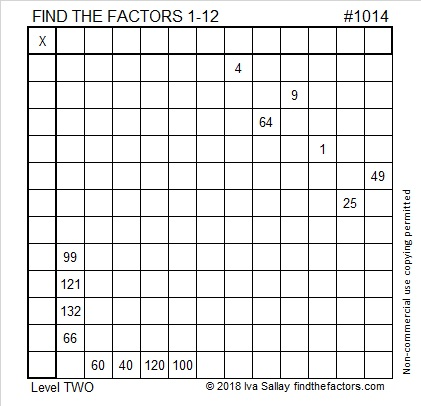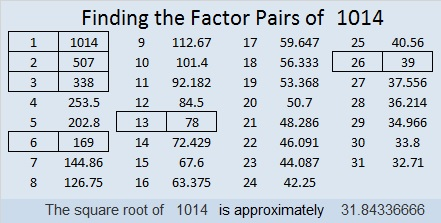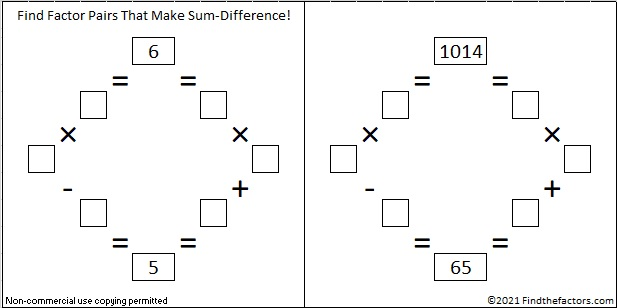# Level 2 and Simplifying √1014

Contents

### Today’s Puzzle:

Have you memorized a basic multiplication table? If you have, then you can solve this puzzle. The numbers being multiplied together aren’t where they are in a regular multiplication table, but you can still easily figure out where they need to go. There is only one solution. I bet you can find it!Print the puzzles or type the solution in this excel file: 12 factors 1012-1018

### Simplifying √1014:

If I wanted to find √1014, I would first check to see if it were divisible by 4 or by 9 because most numbers whose square roots can be simplified are divisible by 4 or by 9 or both.
1014 isn’t divisible by 4 because 14 isn’t divisible by 4.
It isn’t divisible by 9 because 1 + 0 + 1 + 4 = 6, and 6 is not divisible by 9.
However, it is divisible by both 2 and 3 and thus also by 6. Since most people are less likely to make a mistake dividing by 6 in ONE step instead of two, I would make a little division cake and do that division first:Recognizing that 169 is a perfect square, I would then take the square root of everything on the outside of my little cake. (√6)(√169) = 13√6

### Factors of 1014:

• 1014 is a composite number.
• Prime factorization: 1014 = 2 × 3 × 13 × 13, which can be written 1014 = 2 × 3 × 13²
• The exponents in the prime factorization are 1, 1, and 2. Adding one to each and multiplying we get (1 + 1)(1 + 1)(2 + 1) = 2 × 2 × 3 = 12. Therefore 1014 has exactly 12 factors.
• Factors of 1014: 1, 2, 3, 6, 13, 26, 39, 78, 169, 338, 507, 1014
• Factor pairs: 1014 = 1 × 1014, 2 × 507, 3 × 338, 6 × 169, 13 × 78, or 26 × 39,
• Taking the factor pair with the largest square number factor, we get √1014 = (√169)(√6) = 13√6 ≈ 31.84337### Sum-Difference Puzzles:

6 has two factor pairs. One of those pairs adds up to 5, and the other one subtracts to 5. Put the factors in the appropriate boxes in the first puzzle.

1014 has six factor pairs. One of the factor pairs adds up to ­65, and a different one subtracts to 65. If you can identify those factor pairs, then you can solve the second puzzle!The second puzzle is really just the first puzzle in disguise. Why would I say that?

### More about the Number 1014:

Because 13² is one of its factors, 1014 is the hypotenuse of two Pythagorean triples:
714-720-1014 which is 6 times (119-120-169),
390-936-1014 which is (5-12-13) times 78.

1014 looks interesting when written in some other bases:
It’s 600 in BASE 13 because 6(13²) = 6 (169) = 1014,
and 222 in BASE 22 because 2(22²) + 2(22) + 2(1) = 2(484 + 22 + 1) = 2(507) = 1014.

This site uses Akismet to reduce spam. Learn how your comment data is processed.